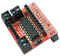There are two ways to program/use your new PicoCon motor controller:

1. Basic python software

• Each motor is directly controlled by two pins, each of which drives one side of the motor
• To make the motor go forwards, you make one pin high and the other pin low
• To make it reverse, you make the first pin low and the second pin high
• For the robot to go forwards both motors should be set to forwards
• Similarly to go backwards, both motors should be set to reverse
• If you set the left motor to forwards and the right motor to backwards, the robot will spin right
• The (physical) pins for the left motor are 19 and 21
• The (physical) pins for the right motor are 24 and 26
• So to make the left motor go forwards, set pin19 high and pin21 low. You can do this in python with: GPIO.output(19, 1)    # pin 19 is left motor A GPIO.output(21, 1)    # pin 21 is left motor B

This is covered in a complete python program picoTest01.py covered below.

2. Using the supplied python library

• You need to import the library at the start of your python program import picocon
• Then you can use the pre-defined functions for moving the motors:
• init(). Initialises GPIO pins, switches motors off, etc
• cleanup(). Sets all motors off and sets GPIO to standard values
• stop(): Stops both motors
• forward(speed): Sets both motors to move forward at speed. 0 <= speed <= 100
• reverse(speed): Sets both motors to reverse at speed. 0 <= speed <= 100
• spinLeft(speed): Sets motors to turn opposite directions at speed. 0 <= speed <= 100
• spinRight(speed): Sets motors to turn opposite directions at speed. 0 <= speed <= 100
• turnForward(leftSpeed, rightSpeed): Moves forwards in an arc by setting different speeds. 0 <= leftSpeed,rightSpeed <= 100
• turnReverse(leftSpeed, rightSpeed): Moves backwards in an arc by setting different speeds. 0 <= leftSpeed,rightSpeed <= 100
• To use these functions, you need to preface them with the name of module, picocon.
• You need to call the function init() at the start of your program to setup all the input and output pins picocon.init()
• You must call the cleanup() function at the end to put all the input and output pins back to where they were picocon.cleanup()
• In between you can do whatever you want, so to make the robot go forwards at a medium speed: picocon.forward(50)
• to spin left at a fast speed picocon.spinLeft(100)